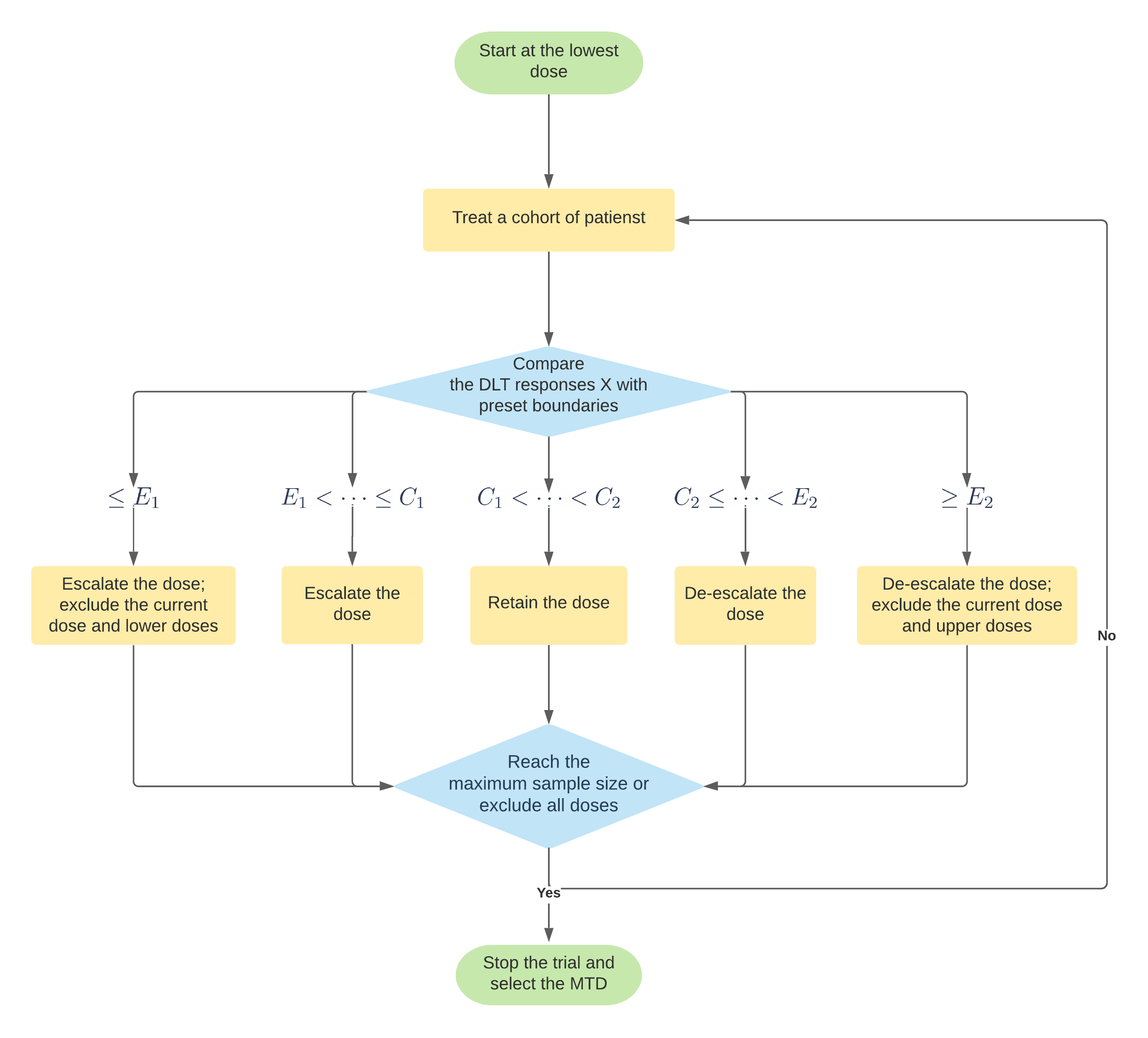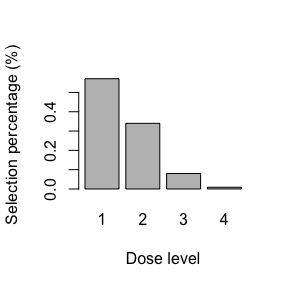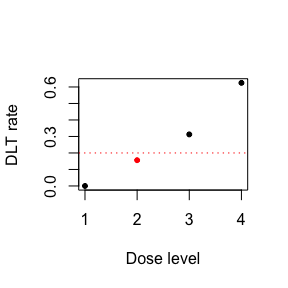# PoP Design

## Introduction

The primary goal of phase I clinical trials is to find the maximum tolerated dose (MTD). To reach this objective, we introduce a new design for phase I clinical trials, the posterior predictive (PoP) design. The PoP design is an innovative model-assisted design that is easy to implement in a manner similar to the traditional 3+3 design, as its decision rules can be pre-tabulated prior to the onset of trial, but is of more flexibility of selecting diverse target toxicity rates and cohort sizes. Moreover, it has satisfactory performance of selecting the true MTD to other more complicated model-based designs. We highlight the desirable properties of the PoP design, such as coherence and consistency. We conduct a numerical simulation study to compare the PoP design to the Bayesian optimal interval design. The results illustrate better performance of the PoP design, with respect to competitive average probabilities of choosing the MTD and lower risk treating patients at overly toxic doses.

## Methods

We assume that there are $$J$$ pre-specified dose levels of the drug of interest. Let $$d_1,d_2,\ldots,d_J$$ denote these dose levels. The dose-limiting toxicity (DLT) is assessed as a binary outcome, experiencing toxicity or not. The true dose toxicity is monotonically increasing as the dose level increases. Let $$\phi$$ be the target toxicity rate and $$\pi_j$$ be the true dose-toxicity of dose level $$d_j$$, for $$j=1,2,\ldots,J$$.

We formulate our hypothesis as: $H_{0j}: \pi_j=\phi \\ H_{1j}: \pi_j\ne\phi$ $$H_{0j}$$ indicates that $$d_j$$ is the desired MTD so that we should stay; $$H_{1j}$$ reflects the current dose is either below or above the MTD so that we should transit to a lower or upper dose level. If the observed toxicity rate is above the target toxicity rate $$\phi$$, we de-escalate the dose; if the observed toxicity rate is below $$\phi$$, we escalate the dose.

With the hypothesis, the predictive Bayes factor comparing $$H_{0j}$$ and $$H_{1j}$$ is given by $$PrBF_{0,1}=$$ where $$x_j$$ is the toxicity response of the ith subject among $$n_j$$ subjects that received dose $$d_j$$, for $$j=1,2,\ldots,J$$.

$$y_j$$ denotes the sum of toxicity response. We assume that $y_j \sim Bin(n_j,\pi_j)$

According to the calibration of the PrBF, a decision rule based on $$PrBF_{0,1}$$ is: 1. If $$PrBF_{0,1}>C(n_j)$$, the evidence is in favor of $$H_{0j}$$ and we need to retain the current dose; 2. Otherwise, we assign the next cohort of patients to an adjacent dose according to the observed DLT $$\hat{\pi}_j = y_j/n_j$$, such as:

1. If $$\hat{\pi}_j < \phi$$, we escalate the dose;

2. If $$\hat{\pi}_j > \phi$$, we de-escalate the dose.

For patient safety and trial efficiency, the PoP design employs a dose exclusion rule. On the one hand, if the PrBF based on the observed DLT indicates a dose is above the MTD with a certain evidence, we exclude the current dose and doses above to avoid treating patients at an overly toxic dose; on the other hand, if the PrBF implies that a dose is substantially below the MTD, we eliminate the current dose and doses below to prevent wasting patients at a subtherapeutic dose. Such a dose exclusion rule is as follow:

If $$PrBF_{0,1}<E(n_j)$$, the evidence is in favor of $$H_{1j}$$ and:

1. If $$\hat{\pi}_j < \phi$$, the current dose is deemed as subtherapeutic and we exclude the current dose and lower doses;

2. If $$\hat{\pi}_j > \phi$$ the current dose is overly toxic and we exclude the current dose and higher doses.

Once all the doses are eliminated from further investigation, the trial is terminated early. The selection of the cut-off value for the dose exclusion is critical for the performance of the PoP design, because it ensure the safety of the patients and efficiency of the design by influencing the early termination rule. The exclusion boundaries in the table above were determined using $$E(n_j)=exp(-1)$$.

## Installation

The R package POPdesign is freely available at the Comprehensive R Archive Network (CRAN). It provides functions for the PoP design in the single-agent dose finding trials. The package can be loaded as follows:

# install.packages("PoPdesign")
library(PoPdesign)

## Obtaining dose escalation and de-escalation boundaries

By specifying the number of cohorts (n.cohort), cohort size and the target DLT rate (target), we can obtain the dose escalation and de-escalation boundaries through the get.boundary.pop() function.

bd <-  get.boundary.pop(n.cohort = 10, cohortsize = 3, target=0.3,
cutoff=exp(1), K=3,cutoff_e=exp(-1))
summary(bd)
#> The decision boundaries are
#>                                              [,1] [,2] [,3] [,4] [,5] [,6] [,7]
#> Number of patients treated                      3    6    9   12   15   18   21
#> Escalation if # of DLT (C1) <=                  0    1    2    3    4    4    5
#> Deescalation if # of DLT (C2) >=                2    3    3    4    5    6    7
#> Subtherapeutic exclusion if # of DLT (E1) <=   NA   NA    0    0    1    1    2
#> Overly toxic exclusion if # of DLT (E2) >=      3    5    6    7    9   10   11
#>                                              [,8] [,9] [,10]
#> Number of patients treated                     24   27    30
#> Escalation if # of DLT (C1) <=                  6    7     8
#> Deescalation if # of DLT (C2) >=                8    9    10
#> Subtherapeutic exclusion if # of DLT (E1) <=    2    3     4
#> Overly toxic exclusion if # of DLT (E2) >=     12   14    15
#>
#> A more completed version of the decision boundaries is given by
#>                                              [,1] [,2] [,3] [,4] [,5] [,6] [,7]
#> Number of patients treated                      1    2    3    4    5    6    7
#> Escalation if # of DLT (C1) <=                 NA    0    0    0    1    1    1
#> Deescalation if # of DLT (C2) >=                1    1    2    2    2    3    3
#> Subtherapeutic exclusion if # of DLT (E1) <=   NA   NA   NA   NA   NA   NA   NA
#> Overly toxic exclusion if # of DLT (E2) >=     NA   NA    3    4    4    5    5
#>                                              [,8] [,9] [,10] [,11] [,12] [,13]
#> Number of patients treated                      8    9    10    11    12    13
#> Escalation if # of DLT (C1) <=                  2    2     2     2     3     3
#> Deescalation if # of DLT (C2) >=                3    3     4     4     4     5
#> Subtherapeutic exclusion if # of DLT (E1) <=    0    0     0     0     0     0
#> Overly toxic exclusion if # of DLT (E2) >=      6    6     7     7     7     8
#>                                              [,14] [,15] [,16] [,17] [,18]
#> Number of patients treated                      14    15    16    17    18
#> Escalation if # of DLT (C1) <=                   3     4     4     4     4
#> Deescalation if # of DLT (C2) >=                 5     5     6     6     6
#> Subtherapeutic exclusion if # of DLT (E1) <=     1     1     1     1     1
#> Overly toxic exclusion if # of DLT (E2) >=       8     9     9    10    10
#>                                              [,19] [,20] [,21] [,22] [,23]
#> Number of patients treated                      19    20    21    22    23
#> Escalation if # of DLT (C1) <=                   5     5     5     6     6
#> Deescalation if # of DLT (C2) >=                 7     7     7     7     8
#> Subtherapeutic exclusion if # of DLT (E1) <=     1     2     2     2     2
#> Overly toxic exclusion if # of DLT (E2) >=      10    11    11    12    12
#>                                              [,24] [,25] [,26] [,27] [,28]
#> Number of patients treated                      24    25    26    27    28
#> Escalation if # of DLT (C1) <=                   6     7     7     7     7
#> Deescalation if # of DLT (C2) >=                 8     8     9     9     9
#> Subtherapeutic exclusion if # of DLT (E1) <=     2     3     3     3     3
#> Overly toxic exclusion if # of DLT (E2) >=      12    13    13    14    14
#>                                              [,29] [,30]
#> Number of patients treated                      29    30
#> Escalation if # of DLT (C1) <=                   8     8
#> Deescalation if # of DLT (C2) >=                10    10
#> Subtherapeutic exclusion if # of DLT (E1) <=     4     4
#> Overly toxic exclusion if # of DLT (E2) >=      14    15

The plot() output includes one flowchart along with the dose escalation/de-escalation table. The flowchart provides detailed information on how to conduct the PoP design. To open the flowchart, please extend the image Viewer window.

plot(bd)
#>   format width height colorspace matte filesize density
#> 1    PNG  2738   2475       sRGB  TRUE   209642 118x118
#>                                              [,1] [,2] [,3] [,4] [,5] [,6] [,7]
#> Number of patients treated                      3    6    9   12   15   18   21
#> Escalation if # of DLT (C1) <=                  0    1    2    3    4    4    5
#> Deescalation if # of DLT (C2) >=                2    3    3    4    5    6    7
#> Subtherapeutic exclusion if # of DLT (E1) <=   NA   NA    0    0    1    1    2
#> Overly toxic exclusion if # of DLT (E2) >=      3    5    6    7    9   10   11
#>                                              [,8] [,9] [,10]
#> Number of patients treated                     24   27    30
#> Escalation if # of DLT (C1) <=                  6    7     8
#> Deescalation if # of DLT (C2) >=                8    9    10
#> Subtherapeutic exclusion if # of DLT (E1) <=    2    3     4
#> Overly toxic exclusion if # of DLT (E2) >=     12   14    15## Simulate operative characteristics

The function get.oc.pop() can be used to obtain the operating characteristics of the PoP design.

oc <- get.oc.pop(target=0.3,n.cohort=10,cohortsize=3,titration=TRUE,
cutoff=TRUE,cutoff_e=exp(-1),
skeleton=c(0.3,0.4,0.5,0.6),n.trial=1000,
risk.cutoff=0.8,earlyterm=TRUE,start=1)

summary(oc) # summarize design operating characteristics
#> selection percentage at each dose level (%):
#> 57.1  34.0  8.0  0.9
#> average number of patients treated at each dose level:
#> 12.0  8.4  4.0  1.1
#> average number of toxicity observed at each dose level: 3.6  3.2  2.0  0.7
#> average number of toxicities: 9.6
#> average number of patients: 25.5
#> percentage of early stopping due to toxicity: 29.1 %
#> risk of underdosing (>80% of patients treated below the MTD): 0.0 %
#> risk of overdosing (>80% of patients treated above the MTD): 16.3 %
plot(oc)## Select the MTD

When the trial is completed, we can choose the MTD with observed data by select.mtd.pop().

n <- c(4, 4, 16, 8, 0)
y <- c(0, 0, 5, 5, 0)
selmtd <- select.mtd.pop(target = 0.2, n.pts=n, n.tox=y)
summary(selmtd)
#> The MTD is dose level  2
#>
#> Dose    Posterior DLT
#> Level     Estimate
#>   1          0
#>   2          0.16
#>   3          0.31
#>   4          0.62
plot(selmtd)